# 連線查詢？子查詢？看這篇文章就行了！

## 一、內連線查詢

##### 1.查詢兩個表的所有資料，以笛卡爾積的形式展現出來

SELECT * FROM 表1，表2；

``select * from t_book;``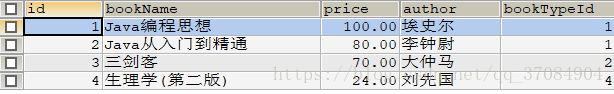``select * from t_booktype;``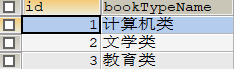``select * from t_book,t_booktype;``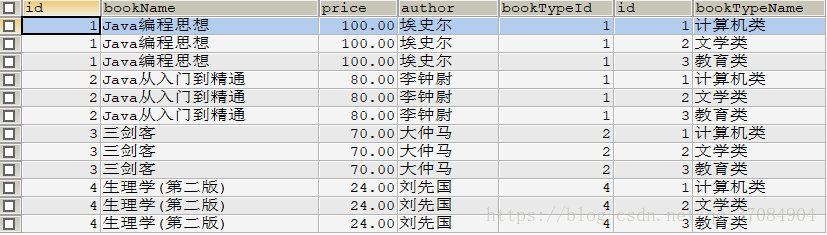##### 2.將兩張表的資料合成一張表（欄位結合）``select * from t_book,t_booktype where t_book.bookTypeId=t_booktype.id;``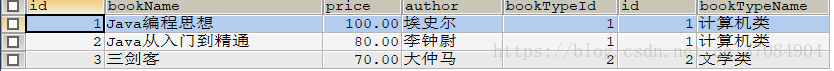``select bookName,price,author,bookTypeName from t_book,t_booktype where t_book.bookTypeId=t_booktype.id;``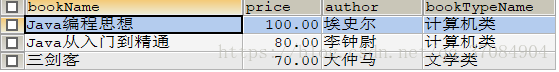``select tb.bookName,tb.price,tb.author,tby.bookTypeName from t_book tb,t_booktype tby where tb.bookTypeId=tby.id;``## 二、外連線查詢

SELECT * FROM 表名1 LEFE|RIGHT JOIN 表名2 ON 表名1.屬性1=表名2.屬性2；

##### 1.左連線查詢：

``select * from t_book left join t_bookType on t_book.Id=t_bookTypeId.id;``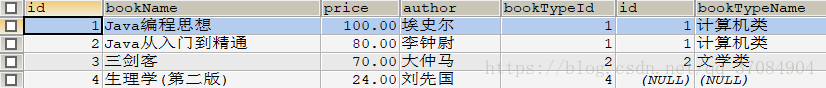##### 2.右連線查詢：

``select * from t_book left join t_bookType on t_book.Id=t_bookTypeId.id;``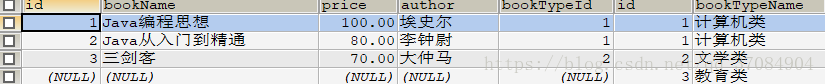## 三、子查詢

##### 1.帶IN關鍵字的子查詢

``select * from t_book where bookTypeId in (select id from t_booktype);``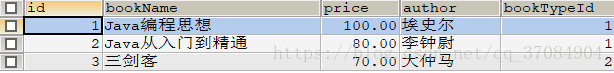``select * from t_book where bookTypeId not in (select id from t_booktype);``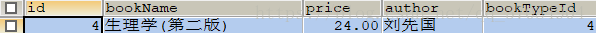##### 2.帶比較運算子的子查詢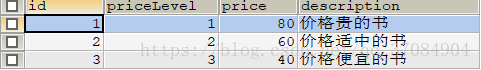``select * from t_book where price >=(select price from t_pricelevel where priceLevel = 1);``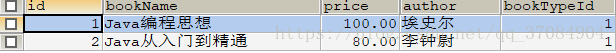##### 3.帶exist關鍵字查詢

``select * from t_book where exists (select * from t_booktype);``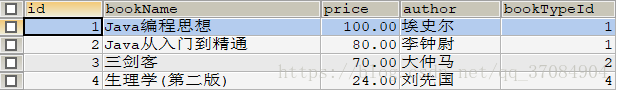##### 4.帶any的關鍵字子查詢

``select * from t_book where price > any (select price from t_pricelevel where priceLevel );``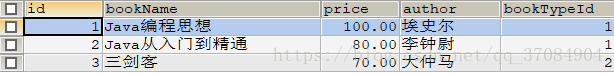##### 5.帶all的關鍵字查詢
``select * from t_book where price> all (select price from t_pricelevel);``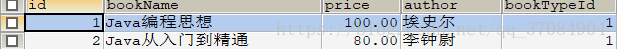t_book表中只有兩條資料大於t_pricelevel表中最大的價格80；

「其他文章」